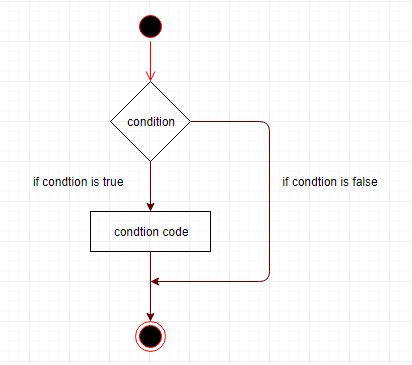if-then语句是IF控制语句中最简单的形式，经常用于决策和更改程序执行的控制流程。

IF语句将条件与关键字THENEND IF所包含的语句序列相关联。如果条件为TRUE，则语句将被执行，如果条件为FALSENULL，则IF语句块不会执行任何操作。

IF-THEN语句的语法是 -

IF condition THEN
S;
END IF;

IF (a <= 20) THEN
c:= c+1;
END IF;DECLARE
a number(2) := 10;
BEGIN
a:= 10;
-- check the boolean condition using if statement
IF( a < 20 ) THEN
-- if condition is true then print the following
dbms_output.put_line('a is less than 20 ' );
END IF;
dbms_output.put_line('value of a is : ' || a);
END;
/

a is less than 20
value of a is : 10

PL/SQL procedure successfully completed.

DECLARE
c_id customers.id%type := 1;
c_sal  customers.salary%type;
BEGIN
SELECT  salary
INTO  c_sal
FROM customers
WHERE id = c_id;
IF (c_sal <= 2000) THEN
UPDATE customers
SET salary =  salary + 1000
WHERE id = c_id;
dbms_output.put_line ('Salary updated');
END IF;
END;
/

Salary updated

PL/SQL procedure successfully completed.